## Example Questions

← Previous 1

### Example Question #1 : Venn Diagrams

In a group of 83 gym members, 51 are taking kickboxing and 25 are taking yoga. Of the students taking kickboxing or yoga, 11 are taking both classes. How many members are not taking either course?

39

24

7

18

18

Explanation:

If 11 people are taking both courses, this means 51-11 or 40 are taking kickboxing only and 25-11 or 14 are taking yoga only. The number of people taking at least one course, therefore, is 40 + 14 + 11 = 65. The 83 members minus the 65 that are taking courses leaves 18 who are not taking any courses.

### Example Question #1 : Venn Diagrams

Doug has a cow farm.  Some of Doug's cows are used for milk, some are used for reproduction and some are used for both. If he has a total of 40 cows and 10 are used only for milk and 3 are used for both milk and reproduction, then how many cows are used for reproduction?

33

27

40

30

13

30

Explanation:

Since we know that only 10 cows are for milk only we must subtract this number from the total amount of cows to get our answer: 40 – 10 = 30 cows. The cows that do both are still used for reproduction, so the correct answer is 30 cows.

### Example Question #1 : Venn Diagrams

All students have to take at least one math class and one language class. Twenty students take calculus, and thirty students take statistics. Fifteen students take Spanish and twenty-five take French. If there are thirty-five students total, what is the maximum number of students taking both two math classes and two language classes.

9

18

5

15

7

5

Explanation:

Totalling the number in math there are 50 students on the rosters of all the math classes. With 35 total students this means that there are 15 students taking 2 math classes. For the language classes there are 40 students on the roster, showing that 5 students are taking 2 language classes. The maximum number of students taking two math classes and two language classes is only as great as the smallest number taking a double math or language class, which is 5 students (limited by the language doubles).

### Example Question #1 : Venn Diagrams

The class of 2034 at Make Believe High School graduated 50 students. 13 students studied only math. 35 students studied English.  30 students studied only 2 subjects. Only 4 students studied writing, it was the third subject for all of them. How many students did not study anything?

10

11

2

0

5

2

Explanation:

The answer is 2. Taking away the 4 writing students, the 13 math-only students, and the remaining 31 English students, we have 2 students remaining.

### Example Question #1 : Data Analysis

In a school of 1250 students, 50% of the students take an art class and 50% of the students take a gym class. If 450 students take neither art nor gym class, then how many students take both art and gym?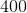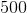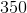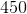Explanation:

You can construct a Venn diagran in which one circle represents art (A), the other represents gym (B), the region of overlap is designated (C), and the number of students not present in either circle is designated (S).

First, it is given that S=450.

50% of students take art and 50% take gym and the total number of students is 1250. 50% of 1250 is 625. Thus, A+C=625 and B+C=625.

Setting them equal to each other we get A+C=B+C.

Subtract C from both sides to get A=B; so the same number of students take art only and gym only.

Now 1250-450= A+B+C=800.

Since A=B we can use substitution to get 2A+C=800.

Finally you can solve the system of equations using the method of your choice (substitution or elimination) to solve the system with A+C=625 and 2A+C=800.

For substitution, we solve for C in the first equation to get C=625-A. Then we substitute this value into the second equation to get 2A+ (625-A)=800. Solve for A to get A=175, so B=175 also. Since A+B+C = 800, C=450.

### Example Question #1 : Venn Diagrams

The universal set is positive counting numbers less than 11.  Set A = {1, 3, 5} and Set B = { 2, 4, 6}.

What is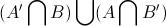?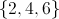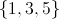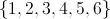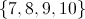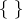Explanation: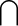means intersection and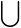means union

U = {1, 2, 3, 4, 5, 6, 7, 8, 9, 10}

A = {1, 3, 5}

A' = {2, 4, 6, 7, 8, 9, 10}

B = {2, 4, 6}

B' = {1, 3, 5, 7, 8, 9, 10}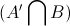= {2, 4, 6}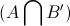= {1, 3, 5}= {1, 2, 3, 4, 5, 6}

### Example Question #1 : Venn Diagrams

Sets P, Q, and R consist of the positive factors of 48, 90, and 56, respectively. If set T = P U (Q ∩ R), which of the following does NOT belong to T?

6

24

3

48

28

28

Explanation:

First, let's find the factors of 48, which will give us all of the elements in P. In order to find the factors of 48, list the pairs of numbers whose product is 48.

The pairs are as follows:

1 and 48; 2 and 24; 3 and 16; 4 and 12; 6 and 8

Therefore the factors of 48 are 1, 2, 3, 4, 6, 8, 12, 16, 24, and 48.

Now we can write P = {1, 2, 3, 4, 6, 8, 12, 16, 24, 48}.

Next, we need to find the factors of 90.

Again list the pairs:

1 and 90; 2 and 45; 3 and 30; 5 and 18; 6 and 15; 9 and 10

Then the factors of 90 are 1, 2, 3, 5, 6, 9, 10, 15, 18, 30, 45, and 90.

Thus, Q = {1, 2, 3, 5, 6, 9, 10, 15, 18, 30, 45, 90}.

Next find the factors of 56:

1 and 56; 2 and 28; 4 and 14; 7 and 8

Set R = {1, 2, 4, 7, 8, 14, 28, 56}

Now, we need to find set T, which is P U (Q ∩ R).

We have to start inside the parantheses with Q ∩ R. The intersection of two sets consists of all of the elements that the two sets have in common. The only elements that Q and R have in common are 1 and 2.

Q ∩ R = {1, 2}

Lastly, we must find P U (Q ∩ R).

The union of two sets consists of any element that is in either of the two sets. Thus, the union of P and Q ∩ R will consist of the elements that are either in P or in Q ∩ R. The following elements are in either P or Q ∩ R:

{1, 2, 3, 4, 6, 8, 12, 16, 24, 48}

Therefore, T = {1, 2, 3, 4, 6, 8, 12, 16, 24, 48}.

The problem asks us to determine which choice does NOT belong to T. The number 28 doesn't belong to T.

### Example Question #1 : Venn Diagrams

Given the Venn diagram below, which of the following does not belong to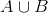?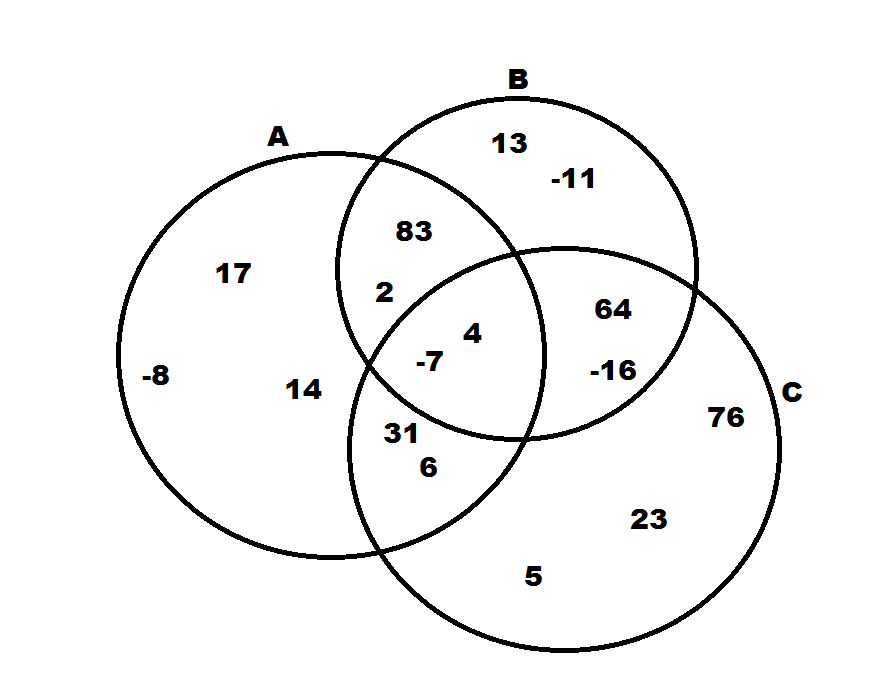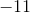Explanation:

The symbol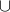stands for the union between two sets.  Therefore,means the set of all numbers that are in either A or B.  Looking at our choices, the only number that isn't in either A, B, or both is 23.

### Example Question #2 : Data Analysis

Sixty high school seniors were polled to see if they were taking history and calculus. A total of 29 students said they were taking calculus, and a total of 50 students said they were taking history. What is the minimum number of students who take both history and calculus?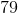Explanation:

We can draw a Venn diagram to see these two sets of students.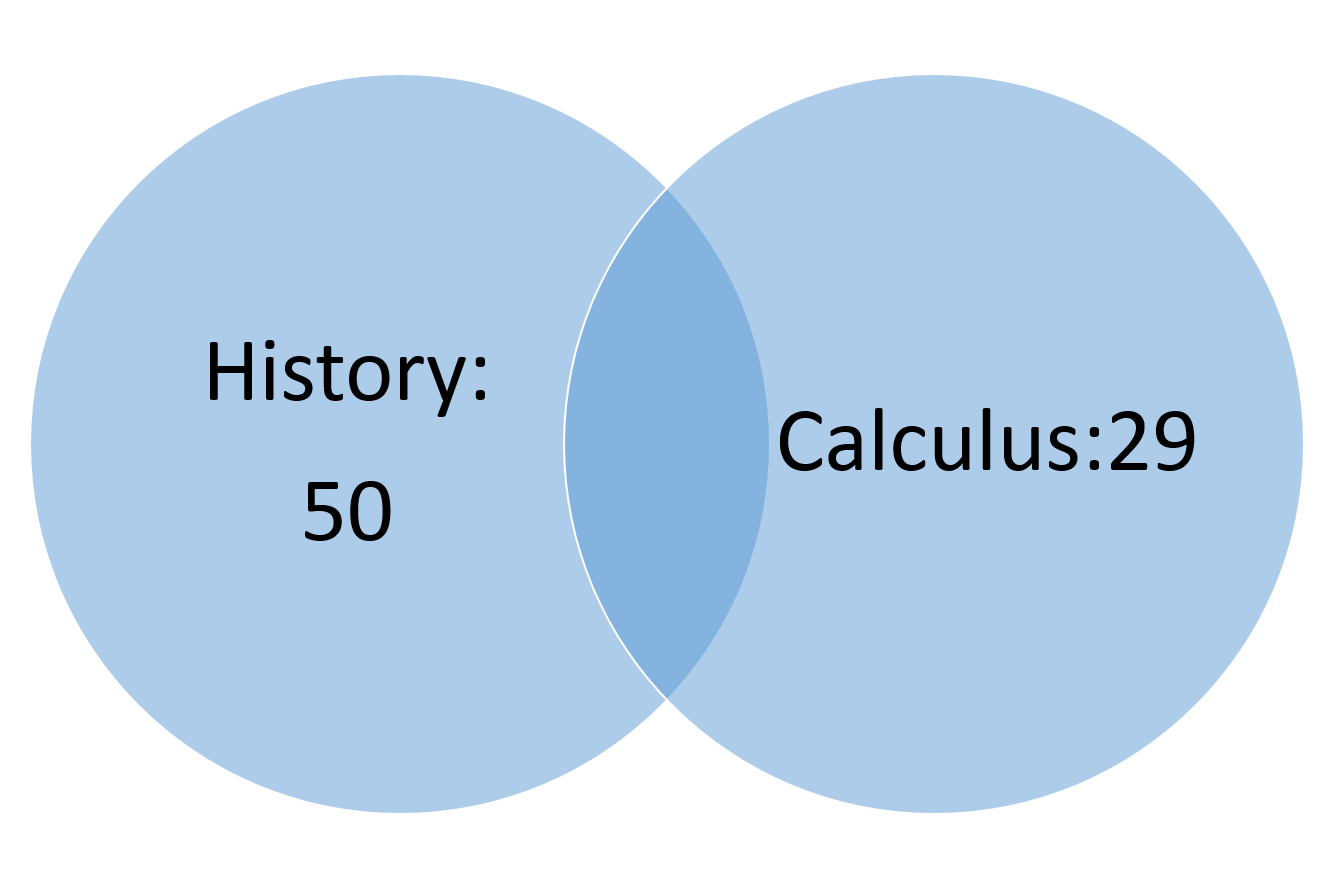We need to find the overlap between these two sets. To find that, add up the total number of students who are taking history and the total number of students who are taking calculus.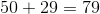Notice that we have more students this way than the total number who were polled. That is because the students who are taking history AND calculus have been double counted. Subtract the total number of students polled to find out how many students were counted twice.

### Example Question #1 : Venn Diagrams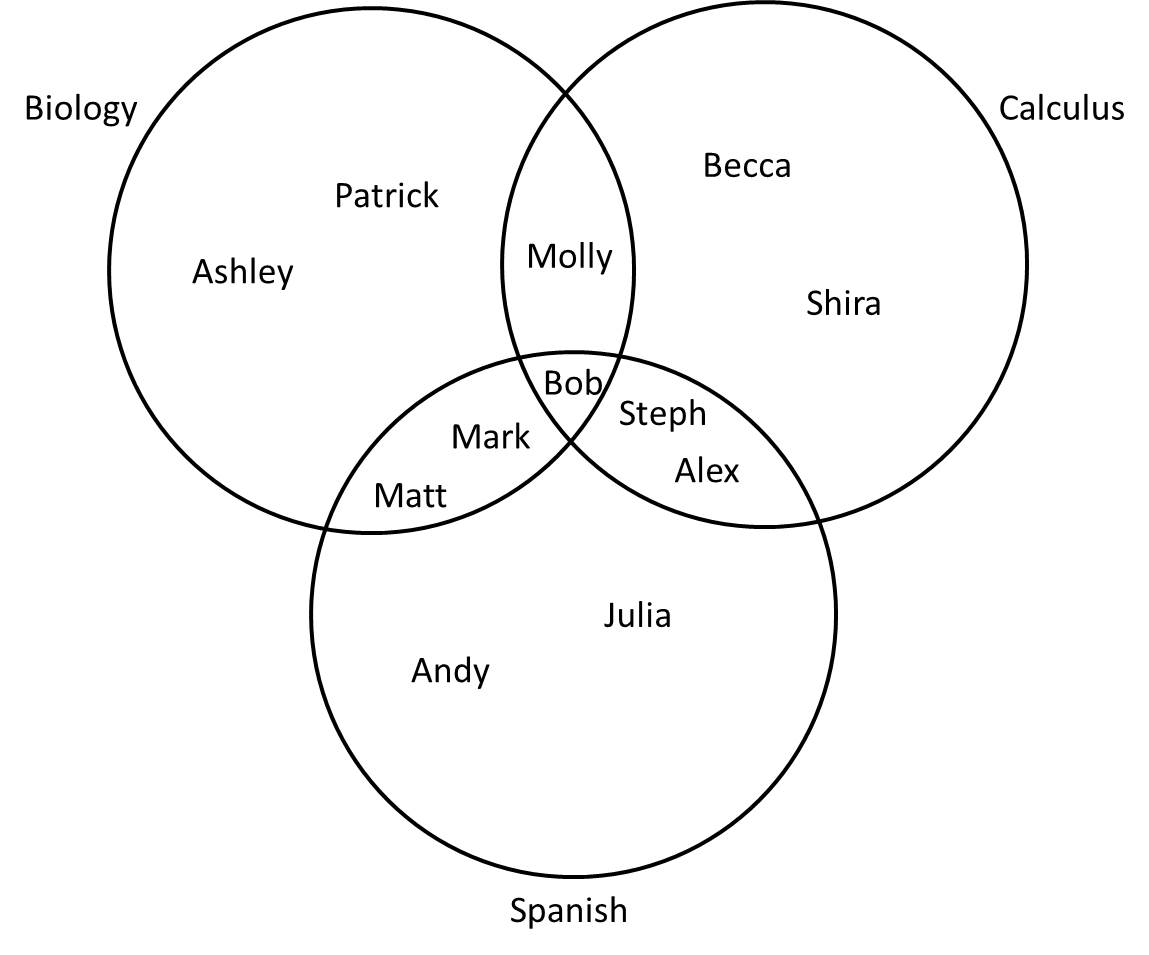A group of high school juniors are taking Biology, Calculus, and Spanish as shown above. Which student is not in the set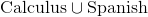?

Molly

Bob

Steph

Andy

Patrick

The notationstands for "union," which refers to everything that is in either set.refers to the group of students taking either Calculus or Spanish (everyone on this diagram except those taking only Biology). From the diagram, Patrick and Ashley are the only students taking neither Calculus nor Spanish, so Patrick is the correct answer.